Search
 Submission Procedure share: |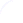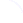available in:   PDF (181 kB) PS (51 kB)

get:
 Similar Docs BibTeX Write a comment
get:

DOI:   10.3217/jucs-004-01-0002

### Dynamical Control of Computations Using the Trapezoidal and Simpson's Rules

Jean-Marie Chesneaux (LIP6 Laboratory, Pierre et Marie Curie University, France)

Fabienne Jézéquel (LIP6 Laboratory, Pierre et Marie Curie University, France)

Abstract:

If In is the approximation of a definite integral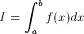with step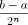using the trapezoidal rule (respectively Simpson's rule), if Ca,b denotes the number of significant digits common to a and b, we show, in this paper, that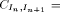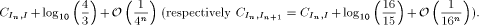According to the previous theorems, using the CADNA library which allows on computers to estimate the round-off error effect on any computed result, we can compute dynamically the optimal value of n to approximate I and we are sure that the exact significant digits of In are in common with the significant digits of I.

Keywords: Simpson's rule, numerical validation, quadrature methods, trapezoidal rule